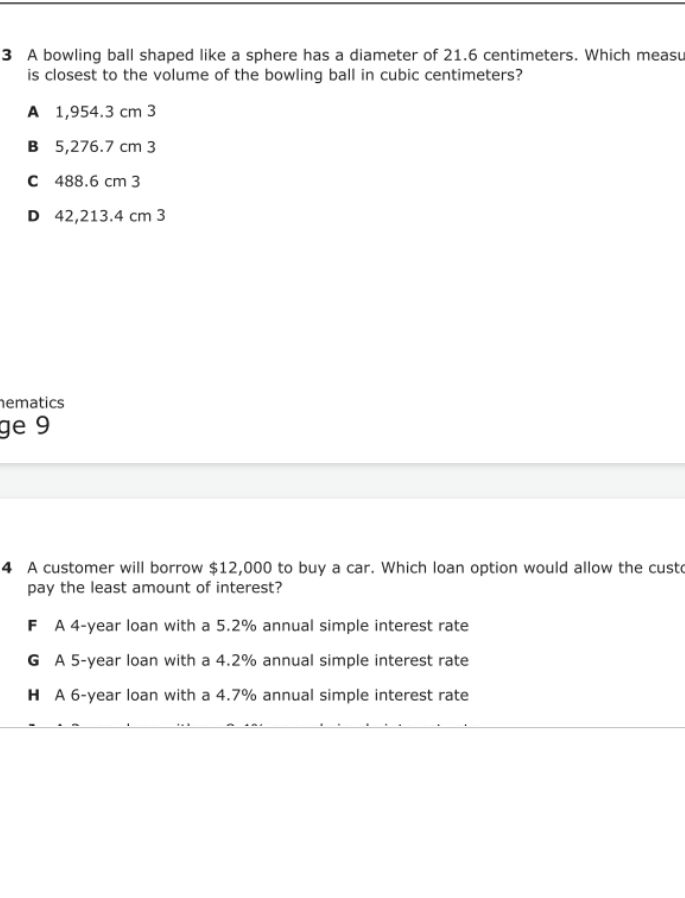### ¿Todavía tienes preguntas de matemáticas?

Pregunte a nuestros tutores expertos
Algebra
Pregunta$$3$$ A bowling ball shaped like a sphere has a diameter of $$21.6$$ centimeters. Which measure is closest to the volume of the bowling ball in cubic centimeters?

$$1,954.3 cm 3$$

$$5,276.7 cm 3$$

$$488.6 cm 3$$

$$42,213.4 cm 3$$